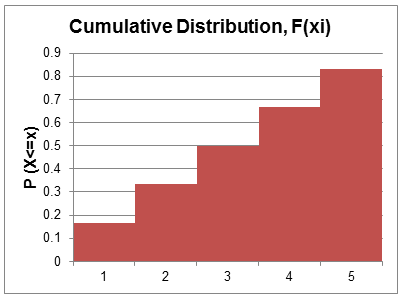# Discrete Uniform Random Variable

A discrete uniform random variable is a discrete random variable for which the probability of each outcome is the same.

Example

The roll of a die is a discrete uniform random variable and has a discrete uniform probability distribution.

The random variable X can take the values X = {1, 2, 3, 4, 5, 6}

Each outcome has a probability of 1/6.

The probability distribution and cumulative distribution functions are shown below:

 xi Probability distribution, P(xi) Cumulative Distribution, F(xi) 1 1/6 1/6 = 0.1667 2 1/6 2/6 = 0.333 3 1/6 3/6 = 0.5 4 1/6 4/6 = 0.667 5 1/6 5/6 = 0.833 6 1/6 6/6 = 1Let’s observe a few values from the above table.

P(3) = 1/6 or 0.1667

F(3) = 0.5

P(2<=X<=5) = 4*1/6 = 0.667

We can generalize this as follows:

P(x) = 1/6 or 0.1667

Cumulative distribution function for any outcome i is F(xi) = i*P(x)

Probability function for a range with k outcomes = k*P(x)

Get our Data Science for Finance Bundle for just $29$51.